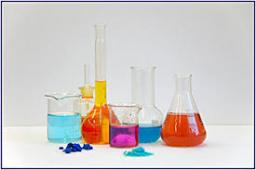# Alcohol 4441

How much 55% alcohol do we need to add to 2 liters of 80% alcohol to produce 60% alcohol? How much is 60% of alcohol made?

a =  8 l
b =  10 l

### Step-by-step explanation:

0.55a+0.80·2=0.6·(a+2)

0.55·a+0.80·2=0.6·(a+2)

0.05a = 0.4

a = 0.4/0.05 = 8

a = 8

Our simple equation calculator calculates it.Did you find an error or inaccuracy? Feel free to write us. Thank you!

Tips for related online calculators
Do you have a system of equations and looking for calculator system of linear equations?